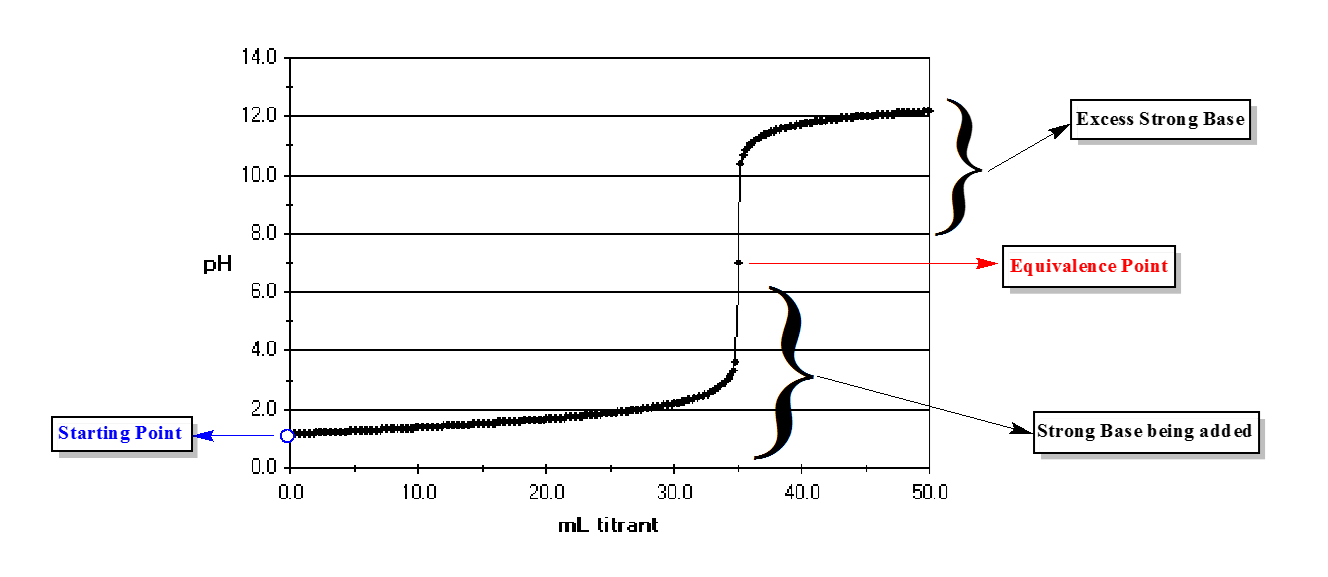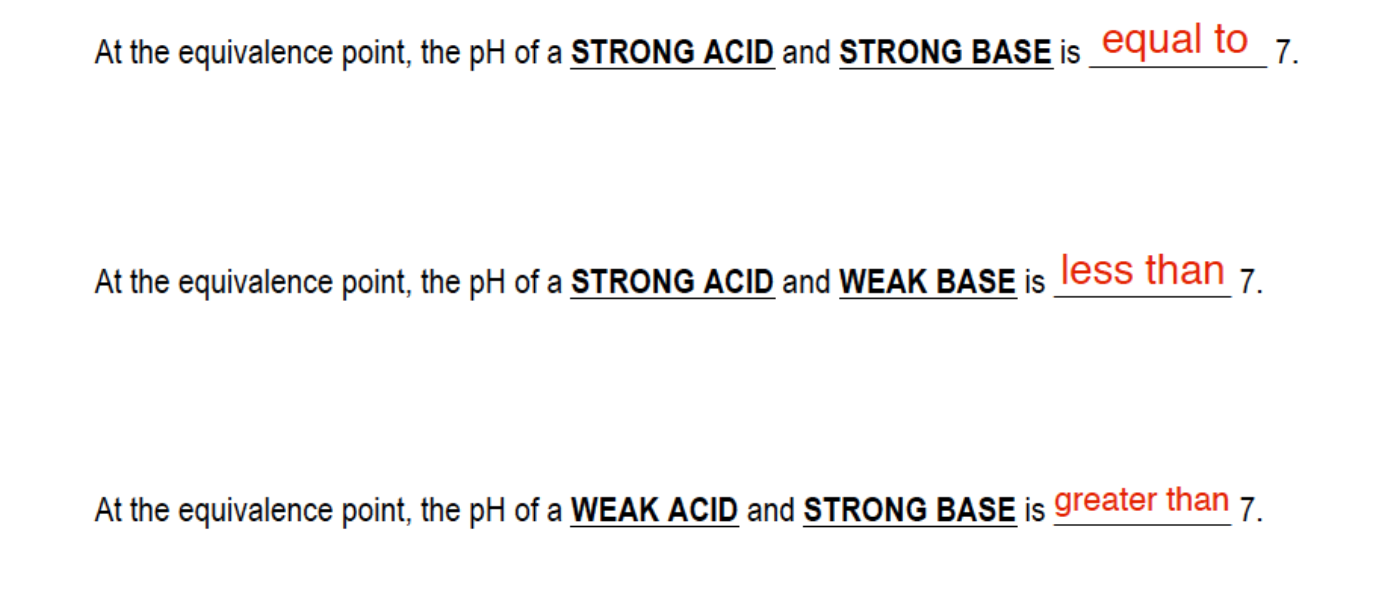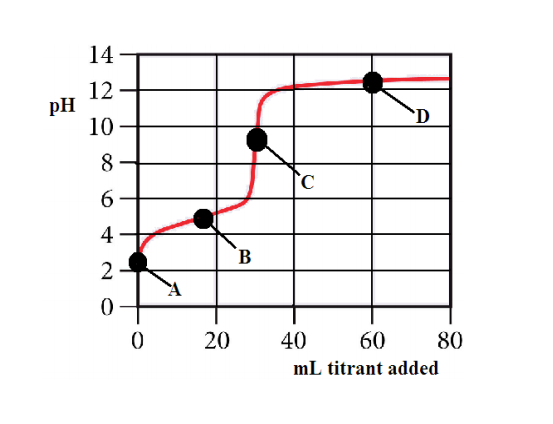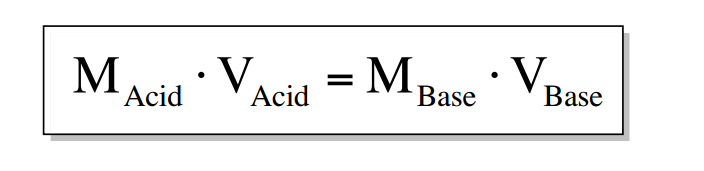Ch.16 - Aqueous Equilibrium WorksheetSee all chapters
 Ch.1 - Intro to General Chemistry 2hrs & 53mins 0% complete Worksheet Ch.2 - Atoms & Elements 2hrs & 49mins 0% complete Worksheet Ch.3 - Chemical Reactions 3hrs & 25mins 0% complete Worksheet BONUS: Lab Techniques and Procedures 1hr & 38mins 0% complete Worksheet BONUS: Mathematical Operations and Functions 47mins 0% complete Worksheet Ch.4 - Chemical Quantities & Aqueous Reactions 3hrs & 55mins 0% complete Worksheet Ch.5 - Gases 3hrs & 47mins 0% complete Worksheet Ch.6 - Thermochemistry 2hrs & 28mins 0% complete Worksheet Ch.7 - Quantum Mechanics 2hrs & 35mins 0% complete Worksheet Ch.8 - Periodic Properties of the Elements 1hr & 57mins 0% complete Worksheet Ch.9 - Bonding & Molecular Structure 2hrs & 5mins 0% complete Worksheet Ch.10 - Molecular Shapes & Valence Bond Theory 1hr & 31mins 0% complete Worksheet Ch.11 - Liquids, Solids & Intermolecular Forces 3hrs & 40mins 0% complete Worksheet Ch.12 - Solutions 2hrs & 17mins 0% complete Worksheet Ch.13 - Chemical Kinetics 2hrs & 22mins 0% complete Worksheet Ch.14 - Chemical Equilibrium 2hrs & 26mins 0% complete Worksheet Ch.15 - Acid and Base Equilibrium 4hrs & 42mins 0% complete Worksheet Ch.16 - Aqueous Equilibrium 3hrs & 48mins 0% complete Worksheet Ch. 17 - Chemical Thermodynamics 1hr & 44mins 0% complete Worksheet Ch.18 - Electrochemistry 2hrs & 58mins 0% complete Worksheet Ch.19 - Nuclear Chemistry 1hr & 33mins 0% complete Worksheet Ch.20 - Organic Chemistry 3hrs 0% complete Worksheet Ch.22 - Chemistry of the Nonmetals 2hrs & 1min 0% complete Worksheet Ch.23 - Transition Metals and Coordination Compounds 1hr & 54mins 0% complete Worksheet

# Acid and Base Titration Curves

See all sections
###### Titration Curves

Titration curves help us to understand the neutralization occurring between an acid and base in order to identify the equivalence point.

###### Equivalence Point

The equivalence point is when the moles of acid and base titrating are equal in amount.

Concept #1: Understanding an Acid–Base Titration Curve.

In a typical titration we begin with an acid or base and slowly add either a Strong Acid or Strong Base.Concept #2: The Equivalence Point.

Depending on the types of acids and bases mixing, at the equivalence point, the pH can be less than, greater than or equal to 7.Example #1: The following questions refer to the titration curve given below.

1) The titration curve shows the titration of

a) a strong acid with a strong base    b) a weak acid with a strong base    c) a strong base with a strong acid    d) a weak base with a strong acid

2) Which point on the titration curve represents a region where a buffer solution has formed?

a) point A      b) point B      c) point C      d) point D

3) Which point on the titration curve represents the equivalence point?

a) point A      b) point B      c) point C      d) point D

4) Which of the following would be the best indicator to use in the titration?

a) erythrosin B, pKa=2.9    b) methyl blue, pKa=5.4    c) bromthymol blue, pKa= 6.8    d) o-cresonphthalein, pKa=9.0An indicator represents a weak acid or base in low concentration that changes color in an acid base titration. An indicator shows us the location of our endpoint, which is located near our equivalence point.

Example #2:  The acid form of an indicator is red and its anion is blue. The Ka value for this indicator is 10 -9. What will be the approximate pH range over which this indicator changes color?
a) 3-5        b) 4-6        c) 5-7        d) 8-10        e) 9-11

An indicator, like a buffer, has an optimal range in which it works most effectively:

pka +/- 1.

Practice: What will be the color of the indicator in the above question in a solution that has a pH of 6?

Example #3: Consider the titration of 100.0 mL of 0.016 M H2SO4 with 0.400 M NaOH at the equivalence point. How many many milliliters of 0.400 M NaOH are required to reach the equivalence point?

If at the equivalence point, the moles of acid equal the moles of base and moles equal molarity multiplied by liters then at the equivalence point we can use the formula:Example #4: Consider the titration of 40.0 mL of 0.0800 M HCl with 0.0160 M Ca(NH2)2.

a) How many milliliters of 0.0160 M Ca(NH2)2 are required to reach the equivalence point?

When dealing with the equivalence point make sure you correctly calculate the molarity for both the acid and base.

Example #5: Consider the titration of 40.0 mL of 0.0800 M HCl with 0.0160 M Ca(NH2)2.

b) What is the pH of this solution?

Practice: Consider the titration of 60.0 mL of 0.200 M H3PO3 solution with 0.350 M potassium hydroxide, KOH solution. How many milliliters of base would be required to reach each of its equivalence points?